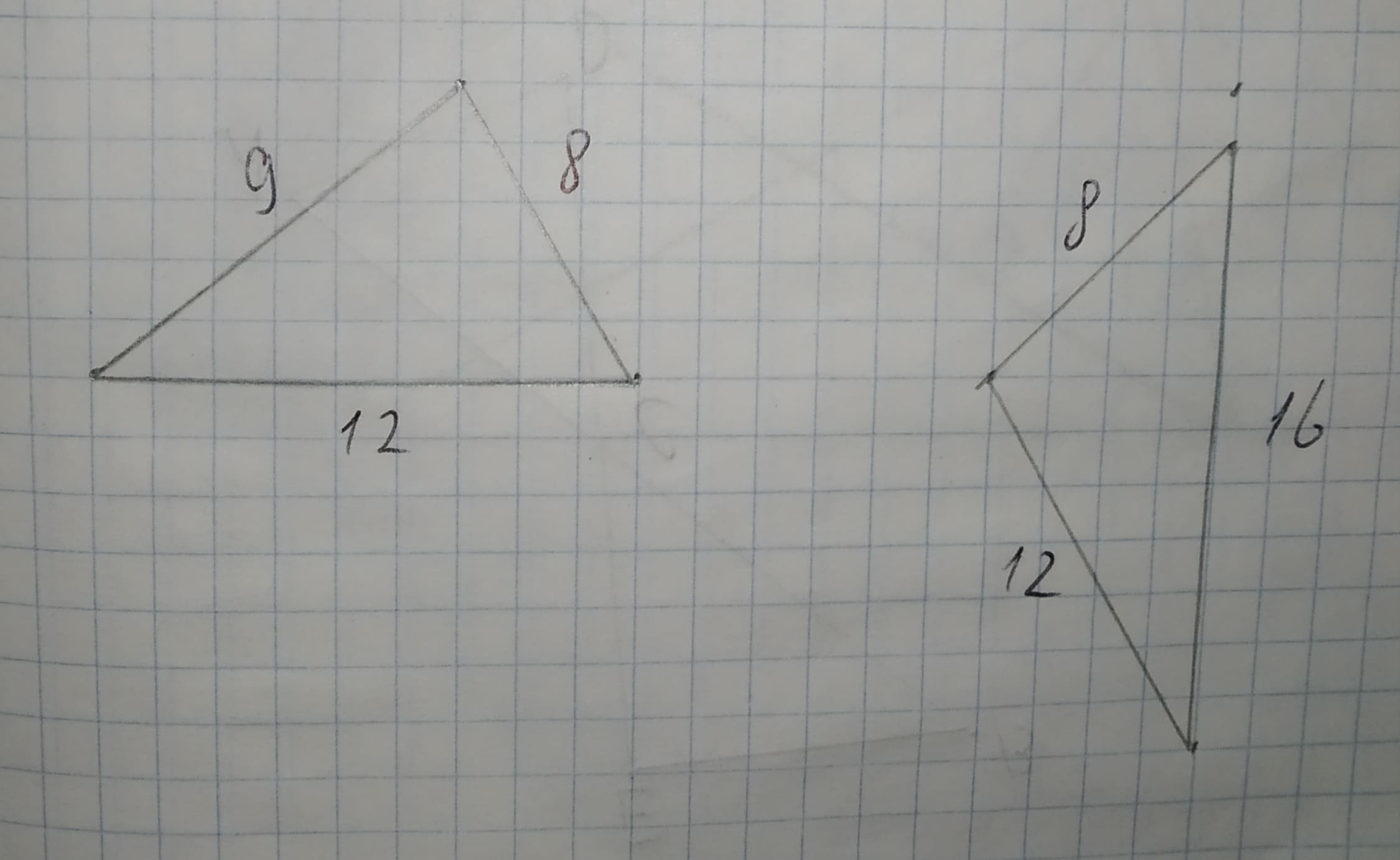# To determine:Given triangles are similar or not, if not give reason.Given information:12210203021.jpgRamsey 2021-02-11 Answered

To determine:Given triangles are similar or not, if not give reason.
Given information:• Questions are typically answered in as fast as 30 minutes

### Plainmath recommends

• Get a detailed answer even on the hardest topics.
• Ask an expert for a step-by-step guidance to learn to do it yourself.okomgcae
In order to find two triangles to be similar all corresponding sides must be proportional. Write ratios corresponding sides in order from least to greatest.
$$\displaystyle{m}=\frac{{{y}_{{2}}-{y}_{{1}}}}{{{x}_{{2}}-{x}_{{1}}}}$$
$$\displaystyle\frac{{{R}{S}}}{{{Y}{W}}}=\frac{{8}}{{8}}={1}$$
$$\displaystyle\frac{{{R}{T}}}{{{Y}{X}}}=\frac{{12}}{{9}}={1.33}$$
$$\displaystyle\frac{{{S}{T}}}{{{W}{X}}}=\frac{{16}}{{12}}={1.33}$$
Since all three pairs of sides are not proportional, $$\displaystyle\triangle{R}{S}{T}\ne\sim{Y}{W}{X}$$ by SSS similarity.